Some colors have names, such as "Red," "Magenta," and "Dark Olive Green." But the most common way to specify a color is to use a hexadecimal value such as CX556B2F. It is not obvious that "Dark Olive Green" and CX556B2F represent the same color, but they do! I like to use color names (when possible) instead of hexadecimal values because the names make the program more readable than the hexadecimal values. For example, a color ramp that is defined by using the names ("DarkSeaGreen" "SandyBrown" "Tomato" "Sienna") is easier to interpret than the equivalent color ramp that is defined by using the hexadecimal values (CX8FBC8F CXF4A460 CXFF6347, CXA0522D).

This article shows how to find a "named color" that is close to any color that you specify. Shakespeare asked, "What's in a name?" To paraphrase his response, this article shows that the name of "Rose" looks just as sweet as CXFF6060 but is easier to use!

### Colors in SAS

When you create a graph in SAS, there are three ways to specify colors: use a pre-defined color name from the SAS registry, use the SAS-naming convention to specify hues, or use hexadecimal values to specify an 8-bit color for the RGB color model. An example of a pre-defined color name is "DarkOliveGreen," an example of a hue-based color is "Dark Moderate Green," and an example of a hexadecimal value is CX556B2F. Each hexadecimal value encodes the three RGB values for the color. For example, the hexadecimal values 55, 6B, and 2F correspond to the decimal integers 85, 107, and 47, so CX556B2F can be thought of as the RGB triplet (85, 107, 47).

In my SAS registry, there are 151 pre-defined color names, whereas there are 2563 = 16.7 million 8-bit RGB colors. Clearly, there are many RGB colors that do not have names! I thought it would be interesting to write a program that finds the closest pre-defined name to any RGB color that you specify. You can think of each color as a three-dimensional point (R, G, B), where R, G, and B are integers and 0 ≤ R,G,B ≤ 255. Thus, the space of all 8-bit colors is a three-dimensional integer lattice. Colors that are close to each other (in the Euclidean metric) have similar shades. Consequently, you can find named color that is closest to another color by using the following steps:

1. Load the pre-defined color names and their RGB values.
2. For any specified hexadecimal value, convert it to an RGB value.
3. In RGB coordinates, find the pre-defined color name that is closest (in the Euclidean metric) to the specified value.

For example, if you specify an unnamed color such as CXE99F62=RGB(244, 107, 53), the program can tell you that "SandyBrown"=RGB(244, 164, 96) is the closest pre-defined color to CXE99F62. If you want to make your program more readable (and don't mind modifying the hues a little), you can replace CXE99F62 with "SandyBrown" in your program.

### Read colors from the SAS registry

For the reference set, I will use the pre-defined colors in the SAS registry, but you could use any other set of names and RGB values. The SAS documentation shows how to use PROC REGISTRY to list the colors in your SAS registry.

The following program modifies the documentation example and writes the registry colors to a temporary text file:

```filename _colors TEMP; /* create a temporary text file */   /* write text file with colors from the registry */ proc registry startat='HKEY_SYSTEM_ROOT\COLORNAMES' list export=_colors; run;   /* In the flat file, the colors look like this: "AliceBlue"= hex: F0,F8,FF "AntiqueWhite"= hex: FA,EB,D7 "Aqua"= hex: 00,FF,FF "Aquamarine"= hex: 7F,FD,D4 "Azure"= hex: F0,FF,FF "Beige"= hex: F5,F5,DC "Bisque"= hex: FF,E4,C4 "Black"= hex: 00,00,00 ... */```

You can use a DATA step to read the registry values and create a SAS data set that contains the names, the hexadecimal representation, and the RGB coordinates for each pre-defined color:

```data RegistryRGB; infile _colors end=eof; /* read from text file; last line sets EOF flag to true */ input; /* read one line at a time into _infile_ */   length ColorName \$32 hex \$8; retain hex "CX000000"; s = _infile_; k = findw(s, 'hex:'); /* does the string 'hex' appear? */ if k then do; /* this line contains a color */ i = findc(s, '=', 2); /* find the second quotation mark (") */ ColorName = substr(s, 2, i-3); /* name is between the quotes */ /* build up the hex value from a comma-delimited value like 'FA,EB,D7' */ substr(hex, 3, 2) = substr(s, k+5 , 2); substr(hex, 5, 2) = substr(s, k+8 , 2); substr(hex, 7, 2) = substr(s, k+11, 2);   R = inputn(substr(hex, 3, 2), "HEX2."); /* get RGB coordinates from hex */ G = inputn(substr(hex, 5, 2), "HEX2."); B = inputn(substr(hex, 7, 2), "HEX2."); end; if k; drop k i s; run;   proc print data=RegistryRGB(obs=8); run;```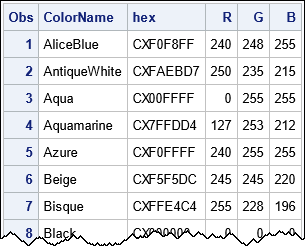The above program works in SAS 9 and also in SAS Viya if you submit the program through SAS Studio. My versions of SAS each have 151 pre-defined colors. The output from PROC PRINT shows that the RegistryRGB data set contains the ColorName, Hex, R, G, and B variables, which describe each pre-defined color.

### Find the closest "named color"

The RegistryRGB data set enables you to answer the following question: Given an RGB color, what "named color" is it closest to?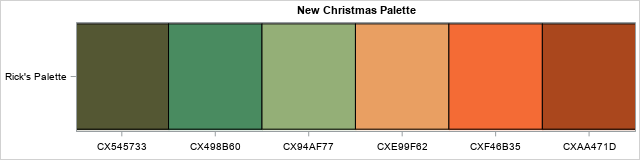For example, in the article "A statistical palette of Christmas colors," I created a palette of colors that had the values {CX545733, CX498B60, CX94AF77, CXE99F62, CXF46B35, CXAA471D}. These colors are shown to the right, but it would be challenging to look solely at the hexadecimal values and know what colors they represent. However, if told you that the colors were close to other colors such as "DarkOliveGreen," "SandyBrown," and "Tomato," you would have a clue about what colors are represented by the hexadecimal values.

The following program uses two functions from previous articles:

```proc iml; /* function to convert an array of colors from hexadecimal to RGB https://blogs.sas.com/content/iml/2014/10/06/hexadecimal-to-rgb.html */ start Hex2RGB(_hex); hex = colvec(_hex); /* convert to column vector */ rgb = j(nrow(hex),3); /* allocate three-column matrix for results */ do i = 1 to nrow(hex); /* for each color, translate hex to decimal */ rgb[i,] = inputn(substr(hex[i], {3 5 7}, 2), "HEX2."); end; return( rgb); finish;   /* Compute indices (row numbers) of k nearest neighbors. INPUT: S an (n x d) data matrix R an (m x d) matrix of reference points k specifies the number of nearest neighbors (k>=1) OUTPUT: idx an (n x k) matrix of row numbers. idx[,j] contains the row numbers (in R) of the j_th closest elements to S dist an (n x k) matrix. dist[,j] contains the distances between S and the j_th closest elements in R https://blogs.sas.com/content/iml/2016/09/28/distance-between-two-group.html */ start PairwiseNearestNbr(idx, dist, S, R, k=1); n = nrow(S); idx = j(n, k, .); dist = j(n, k, .); D = distance(S, R); /* n x m */ do j = 1 to k; dist[,j] = D[ ,><]; /* smallest distance in each row */ idx[,j] = D[ ,>:<]; /* column of smallest distance in each row */ if j < k then do; /* prepare for next closest neighbors */ ndx = sub2ndx(dimension(D), T(1:n)||idx[,j]); D[ndx] = .; /* set elements to missing */ end; end; finish;```

With those two functions defined, the remainder of the program is easy: read the reference RGB colors, define a palette of colors, and find the closest reference color to each specified color.

```/* read the set of reference colors, which have names */ use RegistryRGB; read all var {ColorName hex}; close; RegRGB = Hex2RGB(hex); /* RGB values for the colors in the SAS registry */   /* define the hex values that you want to test */ HaveHex = {CX545733, CX498B60, CX94AF77, CXE99F62, CXF46B35, CXAA471D}; HaveRGB = Hex2RGB(HaveHex); /* convert test values to RGB coordinates */   run PairwiseNearestNbr(ClosestIdx, Dist, HaveRGB, RegRGB); ClosestName = ColorName[ClosestIdx]; /* names of closest reference colors */ ClosestHex = hex[ClosestIdx]; /* hex values for closest reference colors */ print HaveHex ClosestHex ClosestName Dist;```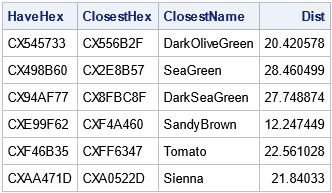The table shows the closest reference color to each specified color. For example, the color CX545733 is closest to the reference color "DarkOliveGreen." How close are they? In three-dimensional RGB coordinates, they are about 20.4 units apart. If you want to see the difference in each coordinate direction, you can print the difference between the RGB values:

```/* how different is each coordinate? */ Diff = HaveRGB - RegRGB[Closestidx,]; print Diff[c={R G B}];```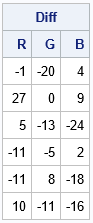You can see that the red and blue coordinates of CX545733 and "DarkOliveGreen" are almost identical. The green coordinates differ by 20 units or about 8%. The "SandyBrown" color is a very good approximation to CXE99F62 because the distance between those colors is about 12.2 units. Every RGB coordinate of "SandyBrown" is within 11 units of the corresponding coordinate of CXE99F62.

You can display both palettes adjacent to each other to compare how well the reference colors approximate the test colors:

```/* visualize the palettes */ ods graphics / width=640px height=160px; k = nrow(HaveHex); run HeatmapDisc(1:k, HaveHex) title="Original Palette" ShowLegend=0 xvalues=HaveHex yvalues="Colors"; run HeatmapDisc(1:k, ClosestHex) title="Nearby Palette of Registry Colors" ShowLegend=0 xvalues=ClosestName yvalues="Colors";```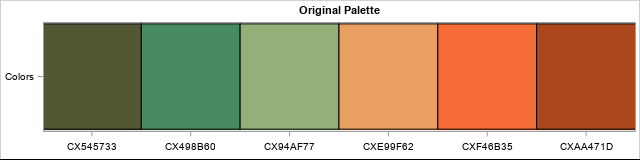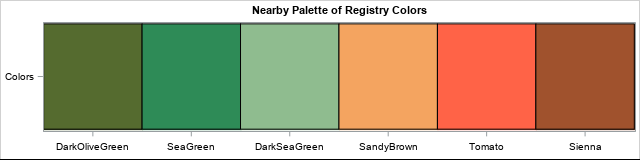The eye can detect small differences in shades, but the overall impression is that the palette of named colors is very similar to the original palette. The palette of named colors is more informative in the sense that people have can visualize "SeaGreen" and "Tomato" without seeing the palette.

### Summary

This article discusses how to create a SAS data set that contains the names and RGB values of a set of "named colors." For this article, I used the named colors in the SAS registry. You can use these named colors as reference colors. Given any other color, you can find the reference color that is closest to the specified color. This enables you to describe the color as being "close to SeaGreen" or "close to SandyBrown," which might help you when you discuss colors with your colleagues.

This article is about approximating colors by using a set of reference colors. If you want to visualize the reference colors themselves, Robert Allison has shown how to display a color swatch for each color in a SAS data set.

The post How to assign a name to a color appeared first on The DO Loop.